# Selina Solutions Concise Maths Class 7 Chapter 3: Fraction (Including Problems) Exercise 3E

Selina Solutions Concise Maths Class 7 Chapter 3 Fraction (Including Problems) Exercise 3E has problems involving fractions, explained in an understandable language, based on the intelligence quotient of students. Students who wish to perform well in the annual exam are advised to solve the exercise problems by referring to the solutions PDF designed by experts. In order to score well in the exams, students can download Selina Solutions Concise Maths Class 7 Chapter 3 Fraction (Including Problems) Exercise 3E free PDF, from the links which are provided here.

## Selina Solutions Concise Maths Class 7 Chapter 3: Fraction (Including Problems) Exercise 3E Download PDF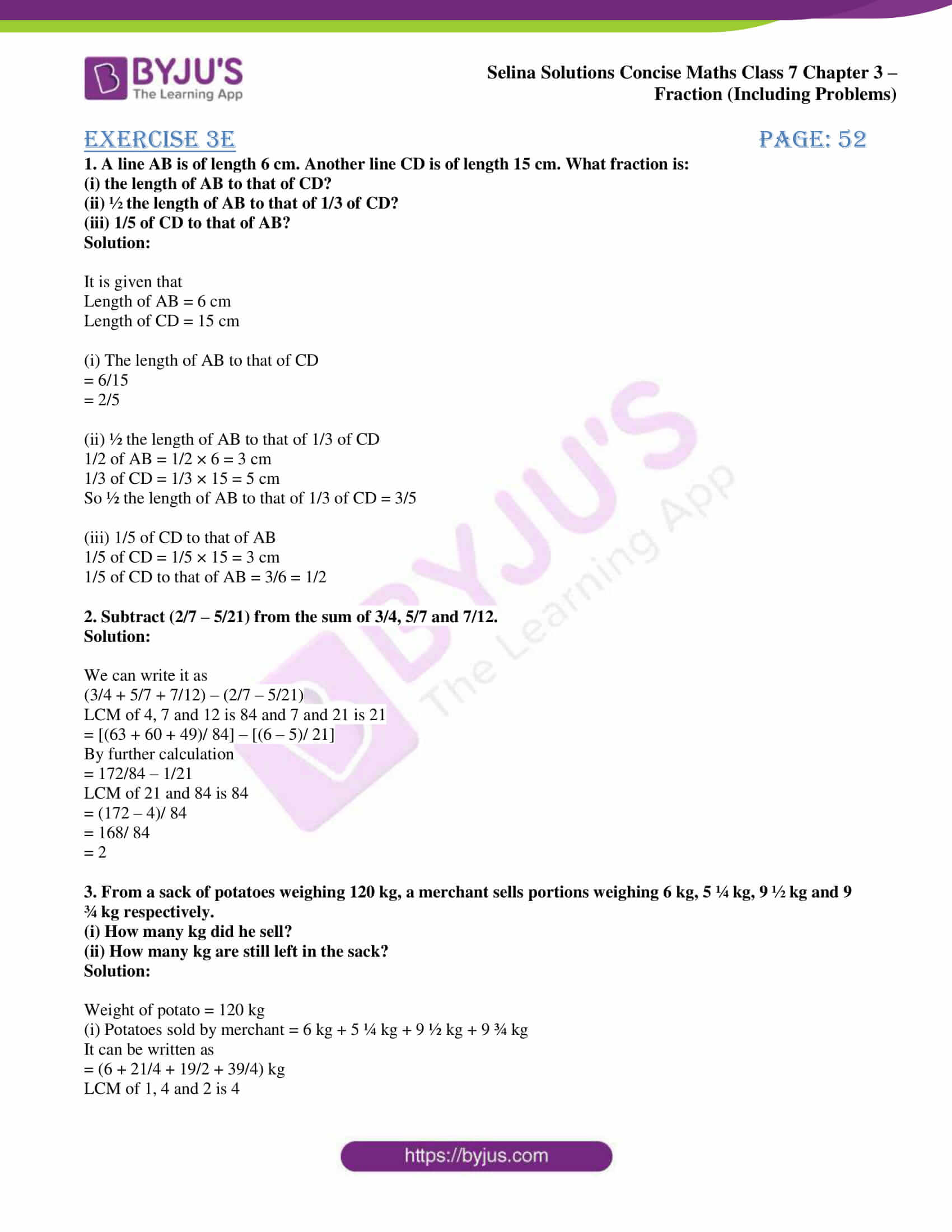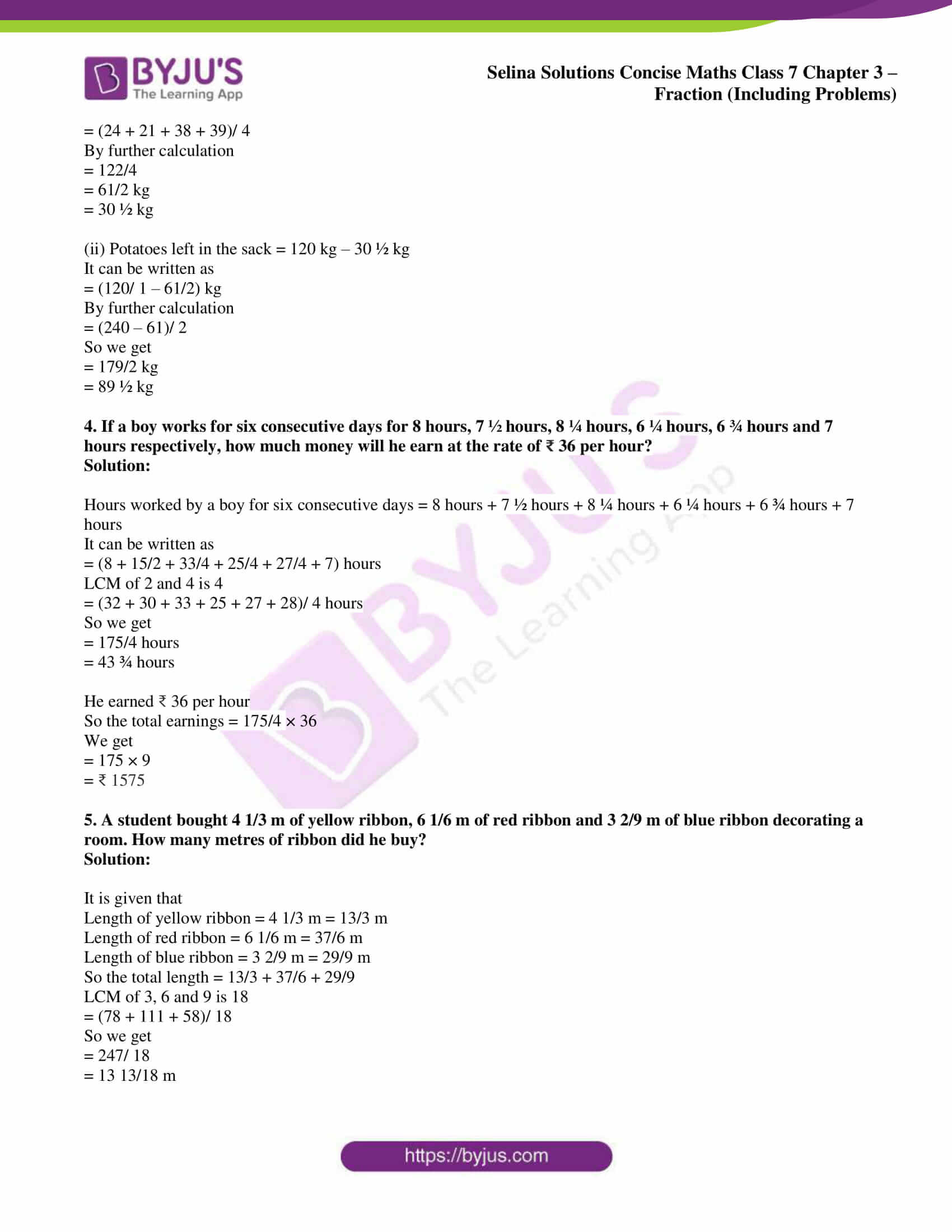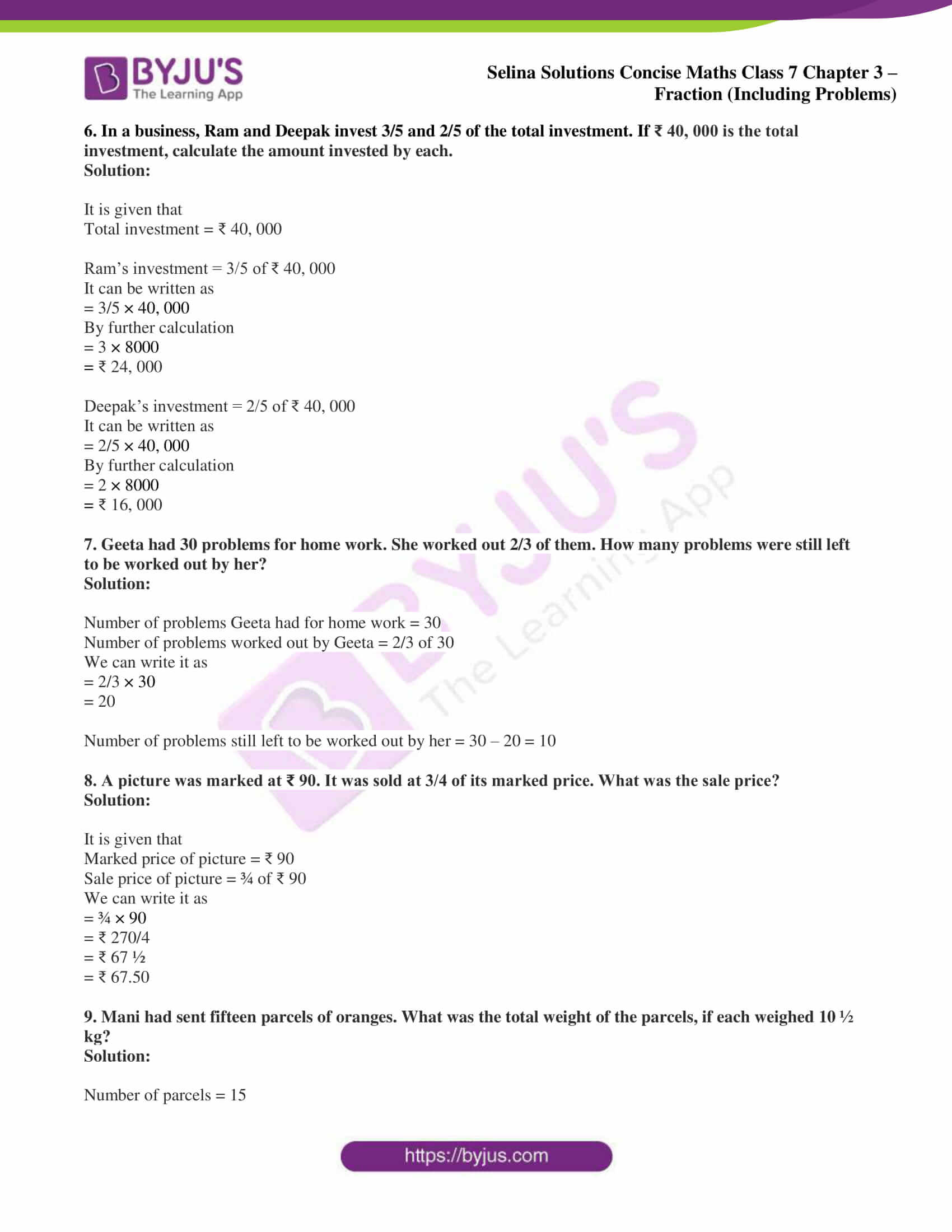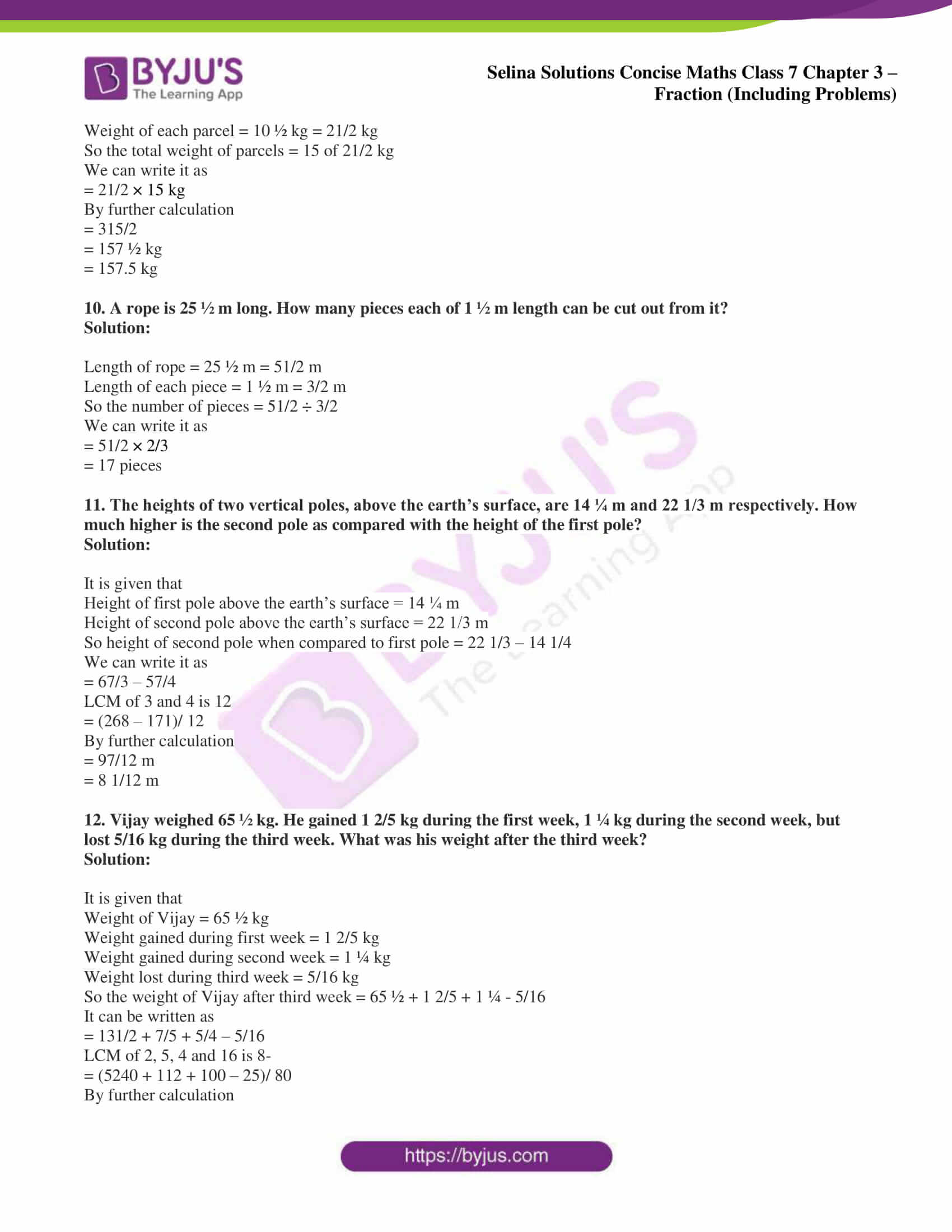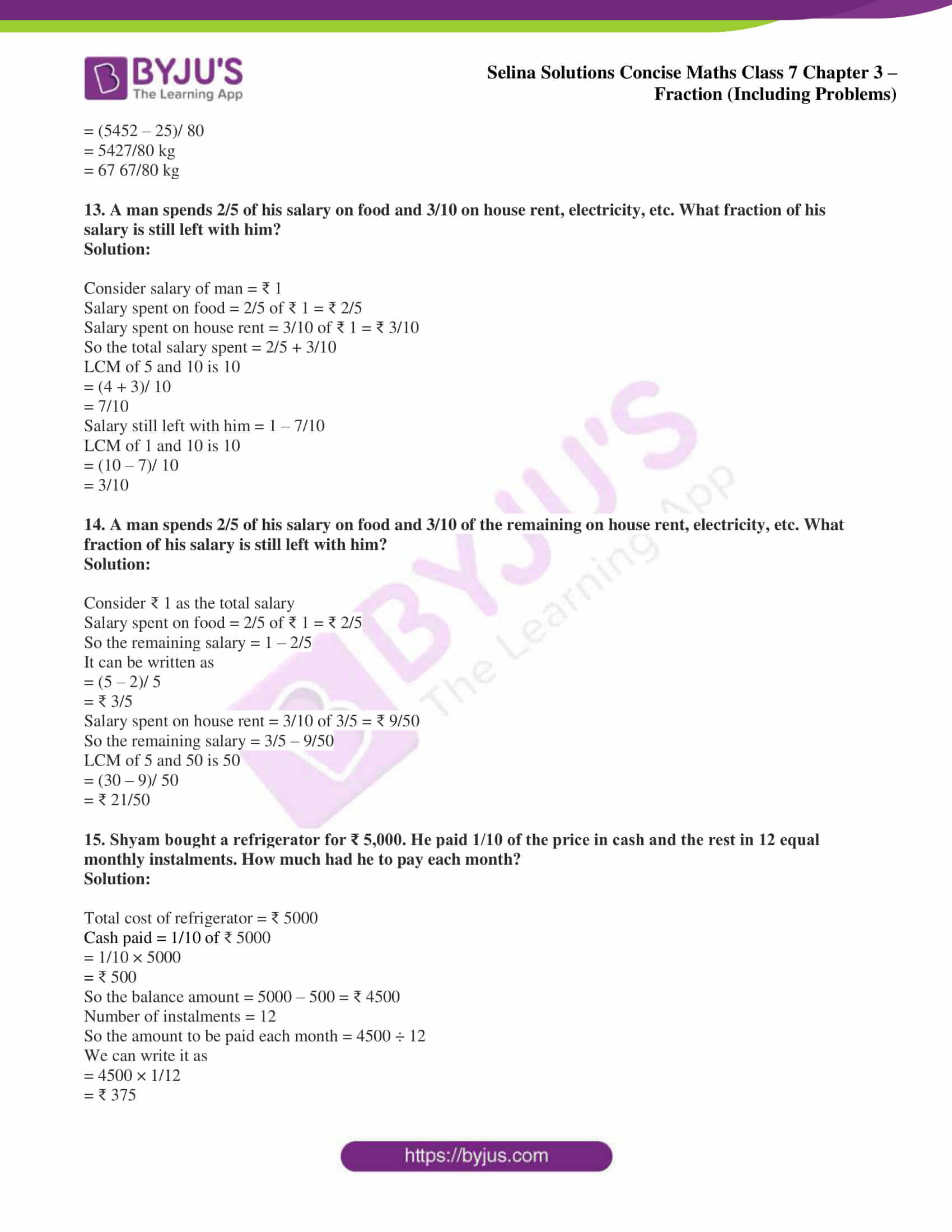### Access Selina Solutions Concise Maths Class 7 Chapter 3: Fraction (Including Problems) Exercise 3E

1. A line AB is of length 6 cm. Another line CD is of length 15 cm. What fraction is:

(i) the length of AB to that of CD?

(ii) ½ the length of AB to that of 1/3 of CD?

(iii) 1/5 of CD to that of AB?

Solution:

It is given that

Length of AB = 6 cm

Length of CD = 15 cm

(i) The length of AB to that of CD

= 6/15

= 2/5

(ii) ½ the length of AB to that of 1/3 of CD

1/2 of AB = 1/2 × 6 = 3 cm

1/3 of CD = 1/3 × 15 = 5 cm

So ½ the length of AB to that of 1/3 of CD = 3/5

(iii) 1/5 of CD to that of AB

1/5 of CD = 1/5 × 15 = 3 cm

1/5 of CD to that of AB = 3/6 = 1/2

2. Subtract (2/7 – 5/21) from the sum of 3/4, 5/7 and 7/12.

Solution:

We can write it as

(3/4 + 5/7 + 7/12) – (2/7 – 5/21)

LCM of 4, 7 and 12 is 84 and 7 and 21 is 21

= [(63 + 60 + 49)/ 84] – [(6 – 5)/ 21]

By further calculation

= 172/84 – 1/21

LCM of 21 and 84 is 84

= (172 – 4)/ 84

= 168/ 84

= 2

3. From a sack of potatoes weighing 120 kg, a merchant sells portions weighing 6 kg, 5 ¼ kg, 9 ½ kg and 9 ¾ kg respectively.

(i) How many kg did he sell?

(ii) How many kg are still left in the sack?

Solution:

Weight of potato = 120 kg

(i) Potatoes sold by merchant = 6 kg + 5 ¼ kg + 9 ½ kg + 9 ¾ kg

It can be written as

= (6 + 21/4 + 19/2 + 39/4) kg

LCM of 1, 4 and 2 is 4

= (24 + 21 + 38 + 39)/ 4

By further calculation

= 122/4

= 61/2 kg

= 30 ½ kg

(ii) Potatoes left in the sack = 120 kg – 30 ½ kg

It can be written as

= (120/ 1 – 61/2) kg

By further calculation

= (240 – 61)/ 2

So we get

= 179/2 kg

= 89 ½ kg

4. If a boy works for six consecutive days for 8 hours, 7 ½ hours, 8 ¼ hours, 6 ¼ hours, 6 ¾ hours and 7 hours respectively, how much money will he earn at the rate of ₹ 36 per hour?

Solution:

Hours worked by a boy for six consecutive days = 8 hours + 7 ½ hours + 8 ¼ hours + 6 ¼ hours + 6 ¾ hours + 7 hours

It can be written as

= (8 + 15/2 + 33/4 + 25/4 + 27/4 + 7) hours

LCM of 2 and 4 is 4

= (32 + 30 + 33 + 25 + 27 + 28)/ 4 hours

So we get

= 175/4 hours

= 43 ¾ hours

He earned ₹ 36 per hour

So the total earnings = 175/4 × 36

We get

= 175 × 9

= ₹ 1575

5. A student bought 4 1/3 m of yellow ribbon, 6 1/6 m of red ribbon and 3 2/9 m of blue ribbon decorating a room. How many metres of ribbon did he buy?

Solution:

It is given that

Length of yellow ribbon = 4 1/3 m = 13/3 m

Length of red ribbon = 6 1/6 m = 37/6 m

Length of blue ribbon = 3 2/9 m = 29/9 m

So the total length = 13/3 + 37/6 + 29/9

LCM of 3, 6 and 9 is 18

= (78 + 111 + 58)/ 18

So we get

= 247/ 18

= 13 13/18 m

6. In a business, Ram and Deepak invest 3/5 and 2/5 of the total investment. If ₹ 40, 000 is the total investment, calculate the amount invested by each.

Solution:

It is given that

Total investment = ₹ 40, 000

Ram’s investment = 3/5 of ₹ 40, 000

It can be written as

= 3/5 × 40, 000

By further calculation

= 3 × 8000

= ₹ 24, 000

Deepak’s investment = 2/5 of ₹ 40, 000

It can be written as

= 2/5 × 40, 000

By further calculation

= 2 × 8000

= ₹ 16, 000

7. Geeta had 30 problems for home work. She worked out 2/3 of them. How many problems were still left to be worked out by her?

Solution:

Number of problems Geeta had for home work = 30

Number of problems worked out by Geeta = 2/3 of 30

We can write it as

= 2/3 × 30

= 20

Number of problems still left to be worked out by her = 30 – 20 = 10

8. A picture was marked at ₹ 90. It was sold at 3/4 of its marked price. What was the sale price?

Solution:

It is given that

Marked price of picture = ₹ 90

Sale price of picture = ¾ of ₹ 90

We can write it as

= ¾ × 90

= ₹ 270/4

= ₹ 67 ½

= ₹ 67.50

9. Mani had sent fifteen parcels of oranges. What was the total weight of the parcels, if each weighed 10 ½ kg?

Solution:

Number of parcels = 15

Weight of each parcel = 10 ½ kg = 21/2 kg

So the total weight of parcels = 15 of 21/2 kg

We can write it as

= 21/2 × 15 kg

By further calculation

= 315/2

= 157 ½ kg

= 157.5 kg

10. A rope is 25 ½ m long. How many pieces each of 1 ½ m length can be cut out from it?

Solution:

Length of rope = 25 ½ m = 51/2 m

Length of each piece = 1 ½ m = 3/2 m

So the number of pieces = 51/2 ÷ 3/2

We can write it as

= 51/2 × 2/3

= 17 pieces

11. The heights of two vertical poles, above the earth’s surface, are 14 ¼ m and 22 1/3 m respectively. How much higher is the second pole as compared with the height of the first pole?

Solution:

It is given that

Height of first pole above the earth’s surface = 14 ¼ m

Height of second pole above the earth’s surface = 22 1/3 m

So height of second pole when compared to first pole = 22 1/3 – 14 1/4

We can write it as

= 67/3 – 57/4

LCM of 3 and 4 is 12

= (268 – 171)/ 12

By further calculation

= 97/12 m

= 8 1/12 m

12. Vijay weighed 65 ½ kg. He gained 1 2/5 kg during the first week, 1 ¼ kg during the second week, but lost 5/16 kg during the third week. What was his weight after the third week?

Solution:

It is given that

Weight of Vijay = 65 ½ kg

Weight gained during first week = 1 2/5 kg

Weight gained during second week = 1 ¼ kg

Weight lost during third week = 5/16 kg

So the weight of Vijay after third week = 65 ½ + 1 2/5 + 1 ¼ – 5/16

It can be written as

= 131/2 + 7/5 + 5/4 – 5/16

LCM of 2, 5, 4 and 16 is 8-

= (5240 + 112 + 100 – 25)/ 80

By further calculation

= (5452 – 25)/ 80

= 5427/80 kg

= 67 67/80 kg

13. A man spends 2/5 of his salary on food and 3/10 on house rent, electricity, etc. What fraction of his salary is still left with him?

Solution:

Consider salary of man = ₹ 1

Salary spent on food = 2/5 of ₹ 1 = ₹ 2/5

Salary spent on house rent = 3/10 of ₹ 1 = ₹ 3/10

So the total salary spent = 2/5 + 3/10

LCM of 5 and 10 is 10

= (4 + 3)/ 10

= 7/10

Salary still left with him = 1 – 7/10

LCM of 1 and 10 is 10

= (10 – 7)/ 10

= 3/10

14. A man spends 2/5 of his salary on food and 3/10 of the remaining on house rent, electricity, etc. What fraction of his salary is still left with him?

Solution:

Consider ₹ 1 as the total salary

Salary spent on food = 2/5 of ₹ 1 = ₹ 2/5

So the remaining salary = 1 – 2/5

It can be written as

= (5 – 2)/ 5

= ₹ 3/5

Salary spent on house rent = 3/10 of 3/5 = ₹ 9/50

So the remaining salary = 3/5 – 9/50

LCM of 5 and 50 is 50

= (30 – 9)/ 50

= ₹ 21/50

15. Shyam bought a refrigerator for ₹ 5,000. He paid 1/10 of the price in cash and the rest in 12 equal monthly instalments. How much had he to pay each month?

Solution:

Total cost of refrigerator = ₹ 5000

Cash paid = 1/10 of ₹ 5000

= 1/10 × 5000

= ₹ 500

So the balance amount = 5000 – 500 = ₹ 4500

Number of instalments = 12

So the amount to be paid each month = 4500 ÷ 12

We can write it as

= 4500 × 1/12

= ₹ 375